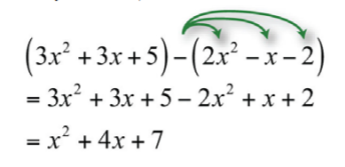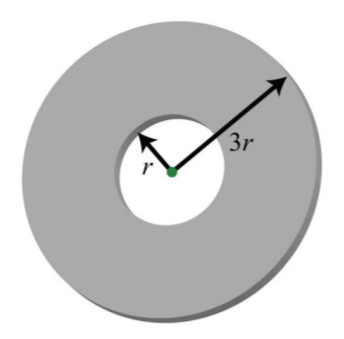$$\newcommand{\id}{\mathrm{id}}$$ $$\newcommand{\Span}{\mathrm{span}}$$ $$\newcommand{\kernel}{\mathrm{null}\,}$$ $$\newcommand{\range}{\mathrm{range}\,}$$ $$\newcommand{\RealPart}{\mathrm{Re}}$$ $$\newcommand{\ImaginaryPart}{\mathrm{Im}}$$ $$\newcommand{\Argument}{\mathrm{Arg}}$$ $$\newcommand{\norm}{\| #1 \|}$$ $$\newcommand{\inner}{\langle #1, #2 \rangle}$$ $$\newcommand{\Span}{\mathrm{span}}$$

# 5.3: Adding and Subtracting Polynomials

$$\newcommand{\vecs}{\overset { \rightharpoonup} {\mathbf{#1}} }$$ $$\newcommand{\vecd}{\overset{-\!-\!\rightharpoonup}{\vphantom{a}\smash {#1}}}$$$$\newcommand{\id}{\mathrm{id}}$$ $$\newcommand{\Span}{\mathrm{span}}$$ $$\newcommand{\kernel}{\mathrm{null}\,}$$ $$\newcommand{\range}{\mathrm{range}\,}$$ $$\newcommand{\RealPart}{\mathrm{Re}}$$ $$\newcommand{\ImaginaryPart}{\mathrm{Im}}$$ $$\newcommand{\Argument}{\mathrm{Arg}}$$ $$\newcommand{\norm}{\| #1 \|}$$ $$\newcommand{\inner}{\langle #1, #2 \rangle}$$ $$\newcommand{\Span}{\mathrm{span}}$$ $$\newcommand{\id}{\mathrm{id}}$$ $$\newcommand{\Span}{\mathrm{span}}$$ $$\newcommand{\kernel}{\mathrm{null}\,}$$ $$\newcommand{\range}{\mathrm{range}\,}$$ $$\newcommand{\RealPart}{\mathrm{Re}}$$ $$\newcommand{\ImaginaryPart}{\mathrm{Im}}$$ $$\newcommand{\Argument}{\mathrm{Arg}}$$ $$\newcommand{\norm}{\| #1 \|}$$ $$\newcommand{\inner}{\langle #1, #2 \rangle}$$ $$\newcommand{\Span}{\mathrm{span}}$$

Learning Objectives

• Subtract polynomials.
• Add and subtract polynomial functions

Recall that we combine like terms, or terms with the same variable part, as a means to simplify expressions. To do this, add the coefficients of the terms to obtain a single term with the same variable part. For example,

$5x^{2}+8x^{2}=13x^{2}$

Notice that the variable part, $$x^{2}$$, does not change. This, in addition to the commutative and associative properties of addition, allows us to add polynomials.

Example $$\PageIndex{1}$$

$$3x+(4x−5)$$.

Solution:

The property $$+(a+b)=a+b$$, which was derived using the distributive property, allows us to remove the parentheses so that we can add like terms.

\begin{aligned} 3x+(4x-5)&=3x+4x-5 \\ &=7x-5 \end{aligned}

$$7x-5$$

Example $$\PageIndex{2}$$

$$(3x^{2}+3x+5)+(2x^{2}−x−2)$$.

Solution:

Remove the parentheses and then combine like terms.

\begin{aligned} (3x^{2}+3x+5)+(2x^{2}-x-2)&=3x^{2}+3x+5+2x^{2}-x-2 \\ &=\color{Cerulean}{\underline{3x^{2}}}\color{black}{+}\color{OliveGreen}{\underline{\underline{3x}}}\color{black}{+5+}\color{Cerulean}{\underline{2x^{2}}}\color{black}{-}\color{OliveGreen}{\underline{\underline{x}}}\color{black}{-2} \\ &=5x^{2}+2x+3 \end{aligned}

$$5x^{2}+2x+3$$

Example $$\PageIndex{3}$$

$$(−5x^{2}y−2xy^{2}+7xy)+(4x^{2}y+7xy^{2}−3xy)$$.

Solution:

Remember that the variable parts have to be exactly the same before we can add the coefficients.

\begin{aligned} &(−5x^{2}y−2xy^{2}+7xy)+(4x^{2}y+7xy^{2}−3xy) \\ &=\color{Cerulean}{\underline{-5x^{2}y}}\color{black}{-}\color{OliveGreen}{\underline{\underline{2xy^{2}}}}\color{black}{+\underline{\underline{\underline{7xy}}}+}\color{Cerulean}{\underline{4x^{2}y}}\color{black}{+}\color{OliveGreen}{\underline{\underline{7xy^{2}}}}\color{black}{-\underline{\underline{\underline{3xy}}}} \\ &=-x^{2}y+5xy^{2}+4xy \end{aligned}

$$-x^{2}y+5xy^{2}+4xy$$

It is common practice to present the terms of the simplified polynomial expression in descending order based on their degree. In other words, we typically present polynomials in standard form, with terms in order from highest to lowest degree.

Example $$\PageIndex{4}$$

$$(a−4a^{3}+a^{5}−8)+(−9a^{5}+a^{4}−7a+5+a^{3})$$.

Solution:

\begin{aligned} &(a−4a^{3}+a^{5}−8)+(−9a^{5}+a^{4}−7a+5+a^{3}) \\ &=a-4a^{3}+a^{5}-8-9a^{5}+a^{4}-7a+5+a^{3} \\ &=-8a^{5}+a^{4}-3a^{3}-6a-3 \end{aligned}

$$-8a^{5}+a^{4}-3a^{3}-6a-3$$

Exercise $$\PageIndex{1}$$

$$(6−5x^{3}+x^{2}−x)+(x^{2}+x+6x^{3}−1)$$.

$$x^{3}+2x^{2}+5$$

## Subtracting Polynomials

When subtracting polynomials, we see that the parentheses become very important. Recall that the distributive property allowed us to derive the following:

$-(a+b)=-a-b$

In other words, when subtracting an algebraic expression, we remove the parentheses by subtracting each term.

Example $$\PageIndex{5}$$

Subtract:

$$10x−(3x+5)$$.

Solution:

Subtract each term within the parentheses and then combine like terms.

\begin{aligned} 10x-(3x+5) &=10x-3x-5 \\ &=7x-5 \end{aligned}

$$7x-5$$

Subtracting a quantity is equivalent to multiplying it by $$−1$$.

Example $$\PageIndex{6}$$

Subtract:

$$(3x^{2}+3x+5)−(2x^{2}−x−2)$$.

Solution:

Distribute the $$−1$$, remove the parentheses, and then combine like terms.Figure $$\PageIndex{1}$$

$$x^{2}+4x+7$$

Multiplying the terms of a polynomial by $$−1$$ changes all the signs.

Example $$\PageIndex{7}$$

Subtract:

$$(−5x^{3}−2x^{2}+7)−(4x^{3}+7x^{2}−3x+2)$$.

Solution:

Distribute the $$−1$$, remove the parentheses, and then combine like terms.

\begin{aligned} &(−5x^{3}−2x^{2}+7)−(4x^{3}+7x^{2}−3x+2) \\ &=-5x^{3}-2x^{2}+7-4x^{3}-7x^{2}+3x-2 \\ &=-9x^{3}-9x^{2}+3x+5 \end{aligned}

$$-9x^{3}-9x^{2}+3x+5$$

Example $$\PageIndex{8}$$

Subtract $$6x^{2}−3x−1$$ from $$2x^{2}+5x−2$$.

Solution

Since subtraction is not commutative, we must take care to set up the difference correctly. First, write the quantity $$(2x^{2}+5x−2)$$; from this, subtract the quantity $$(6x^{2}−3x−1)$$.

\begin{aligned} &(2x^{2}+5x-2)-(6x^{2}-3x-1) \\ &=2x^{2}+5x-2-6x^{2}+3x+1 \\ &=-4x^{2}+8x-1 \end{aligned}

$$-4x^{2}+8x-1$$

Example $$\PageIndex{9}$$

Simplify:

$$(2x^{2}−3x+5)−(x^{2}−3x+1)+(5x^{2}−4x−8)$$.

Solution:

Apply the distributive property, remove the parentheses, and then combine like terms.

\begin{aligned} &(2x^{2}−3x+5)−(x^{2}−3x+1)+(5x^{2}−4x−8) \\ &=2x^{2}-3x+5-x^{2}+3x-1+5x^{2}-4x-8 \\ &=6x^{2}-4x-4 \end{aligned}

$$6x^{2}-4x-4$$

Exercise $$\PageIndex{2}$$

Subtract:

$$(8x^{2}y−5xy^{2}+6)−(x^{2}y+2xy^{2}−1)$$.

$$7x^{2}y-7xy^{2}+7$$

## Adding and Subtracting Polynomial Functions

We use function notation to indicate addition and subtraction of functions as follows:

Addition of functions: $$(f+g)(x)=f(x)+g(x)$$ $$(f-g)(x)=f(x)-g(x)$$

When using function notation, be careful to group the entire function and add or subtract accordingly.

Example $$\PageIndex{10}$$

Calculate:

$$(f+g)(x)$$, given $$f(x)=−x^{2}−3x+5$$ and $$g(x)=3x^{2}+2x+1$$.

Solution:

The notation $$(f+g)(x)$$ indicates that you should add the functions $$f(x)+g(x)$$ and collect like terms.

\begin{aligned} f(x)+g(x)&=(-x^{2}-3x+5)+(3x^{2}+2x+1) \\ &=-x^{2}-3x+5+3x^{2}+2x+1 \\ &=2x^{2}-x+6 \end{aligned}

$$(f+g)(x) = 2x^{2}-x+6$$

Example $$\PageIndex{11}$$

Calculate:

$$(f−g)(x)$$, given $$f(x)=2x−3$$ and $$g(x)=−2x^{2}+2x+5$$.

Solution:

The notation $$(f−g)(x)$$ indicates that you should subtract the functions $$f(x)−g(x)$$:

\begin{aligned} f(x)-g(x) &=(2x-3)-(-2x^{2}+2x+5) \\ &=2x-3+2x^{2}-2x-5 \\ &=2x^{2}-8 \end{aligned}

$$(f-g)(x)=2x^{2}-8$$

We may be asked to evaluate the sum or difference of two functions. We have the option to first find the sum or difference and use the resulting function to evaluate for the given variable, or to first evaluate each function and then find the sum or difference.

Example $$\PageIndex{12}$$

Calculate:

$$(f−g)(5)$$, given $$f(x)=x^{2}+x−7$$ and $$g(x)=4x+10$$.

Solution:

First, find $$(f−g)(x)=f(x)−g(x)$$.

\begin{aligned} f(x)-g(x) & =(x^{2}+x-7)-(4x+10) \\ &=x^{2}+x-7-4x-10 \\ &=x^{2}-3x-17 \end{aligned}

Therefore,

$$(f-g)(x)=x^{2}-3x-17$$

Next, substitute $$5$$ for the variable $$x$$.

\begin{aligned} (f-g)(\color{OliveGreen}{5}\color{black}{)}&=(\color{OliveGreen}{5}\color{black}{)^{2}-3(}\color{OliveGreen}{5}\color{black}{)-17} \\ &=25-15-17 \\ &=-7 \end{aligned}

$$(f-g)(5)=-7$$

Alternate Solution:

Since $$(f−g)(5)=f(5)−g(5)$$, we can find $$f(5)$$ and $$g(5)$$ and then subtract the results.

$$\begin{array}{c|c} {f(x)=x^{2}+x-7}&{g(x)=4x+10}\\{f(\color{OliveGreen}{5}\color{black}{)=(}\color{OliveGreen}{5}\color{black}{)^{2}+(}\color{OliveGreen}{5}\color{black}{)-7}}&{g(\color{OliveGreen}{5}\color{black}{)=4(}\color{OliveGreen}{5}\color{black}{)+10}}\\{=25+5-7}&{=20+10}\\{=23}&{=30} \end{array}$$

Therefore, we have

\begin{aligned} (f-g)(5)&=f(5)-g(5) \\ &=23-30 \\ &=-7 \end{aligned}

$$(f-g)(5)=-7$$

## Key Takeaways

• When adding polynomials, remove the associated parentheses and then combine like terms.
• When subtracting polynomials, distribute the $$−1$$ and subtract all the terms before removing the parentheses and combining like terms.
• The notation $$(f+g)(x)$$ indicates that you add the functions.
• The notation $$(f−g)(x)$$ indicates that you subtract the functions

Exercise $$\PageIndex{3}$$ Addition of Polynomials

1. $$(2x+1)+(−x+7)$$
2. $$(−6x+5)+(3x−1)$$
3. $$(\frac{2}{3}x+\frac{1}{2})+(\frac{1}{3}x−2)$$
4. $$(\frac{1}{3}x−\frac{3}{4})+(\frac{5}{6}x+\frac{1}{8})$$
5. $$(2x+1)+(x−3)+(5x−2)$$
6. $$(2x−8)+(−3x^{2}+7x−5)$$
7. $$(x^{2}−3x+7)+(3x^{2}−8x−5)$$
8. $$(−5x^{2}−1+x)+(−x+7x^{2}−9)$$
9. $$(\frac{1}{2}x^{2}−\frac{1}{3}x+\frac{1}{6})+(−\frac{3}{2}x^{2}+\frac{2}{3}x−1)$$
10. $$(−\frac{3}{5}x2+\frac{1}{4}x−6)+(2x^{2}−\frac{3}{8}x+\frac{5}{2})$$
11. $$(x^{2}+5)+(3x^{2}−2x+1)+(x^{2}+x−3)$$
12. $$(a^{3}−a^{2}+a−8)+(a^{3}+a^{2}+6a−2)$$
13. $$(a^{3}−8)+(−3a^{3}+5a^{2}−2)$$
14. $$(4a^{5}+5a^{3}−a)+(3a^{4}−2a^{2}+7)$$
15. $$(2x^{2}+5x−12)+(7x−5)$$
16. $$(3x+5)+(x^{2}−x+1)+(x^{3}+2x^{2}−3x+6)$$
17. $$(6x^{5}−7x^{3}+x^{2}−15)+(x^{4}+2x^{3}−6x+12)$$
18. $$(1+7x−5x^{3}+4x^{4})+(−3x^{3}+5−x^{2}+x)$$
19. $$(x^{2}y^{2}−7xy+7)+(4x^{2}y^{2}−3xy−8)$$
20. $$(x^{2}+xy−y^{2})+(7x^{2}−5xy+2y^{2})$$
21. $$(2x^{2}+3xy−7y^{2})+(−5x^{2}−3xy+8y^{2})$$
22. $$(a^{2}b^{2}−100)+(2a^{2}b^{2}−3ab+20)$$
23. $$(ab^{2}−3a^{2}b+ab−3)+(−2a^{2}b+ab^{2}−7ab−1)$$
24. $$(10a^{2}b−7ab+8ab^{2})+(6a^{2}b−ab+5ab^{2})$$
25. Find the sum of $$2x+8$$ and $$7x−1$$.
26. Find the sum of $$13x−15$$ and $$16x+110$$.
27. Find the sum of $$x^{2}−10x+8$$ and $$5x^{2}−2x−6$$.
28. Find the sum of $$a^{2}−5a+10$$ and $$−9a^{2}+7a−11$$.
29. Find the sum of $$x^{2}y^{2}−xy+6$$ and $$x^{2}y^{2}+xy−7$$.
30. Find the sum of $$x^{2}−9xy+7y^{2}$$ and $$−3x^{2}−3xy+7y^{2}$$.

1. $$x+8$$

3. $$x−\frac{3}{2}$$

5. $$8x−4$$

7. $$4x^{2}−11x+2$$

9. $$−x^{2}+\frac{1}{3}x−\frac{5}{6}$$

11. $$5x^{2}−x+3$$

13. $$−2a^{3}+5a^{2}−10$$

15. $$2x^{2}+12x−17$$

17. $$6x^{5}+x^{4}−5x^{3}+x^{2}−6x−3$$

19. $$5x^{2}y^{2}−10xy−1$$

21. $$−3x^{2}+y^{2}$$

23. $$−5a^{2}b+2ab^{2}−6ab−4$$

25. $$9x+7$$

27. $$6x^{2}−12x+2$$

29. $$2x^{2}y^{2}−1$$

Exercise $$\PageIndex{4}$$ Subtraction of Polynomials

Subtract.

1. $$(5x−3)−(2x−1)$$
2. $$(−4x+1)−(7x+10)$$
3. $$(\frac{1}{4}x−\frac{3}{4})−(\frac{3}{4}x+\frac{1}{8})$$
4. $$(−\frac{3}{5}x+\frac{3}{7})−(\frac{2}{5}x−\frac{3}{2})$$
5. $$(x^{2}+7x−5)−(4x^{2}−5x+1)$$
6. $$(−6x^{2}+3x−12)−(−6x^{2}+3x−12)$$
7. $$(−3x^{3}+4x−8)−(−x^{2}+4x+10)$$
8. $$(\frac{1}{2}x^{2}+\frac{1}{3}x−\frac{3}{4})−(\frac{3}{2}x^{2}−\frac{1}{6}x+\frac{1}{2})$$
9. $$(\frac{5}{9}x^{2}+\frac{1}{5}x−\frac{1}{3})−(\frac{1}{3}x^{2}+\frac{3}{10}x+\frac{5}{9})$$
10. $$(a^{3}−4a^{2}+3a−7)−(7a^{3}−2a^{2}−6a+9)$$
11. $$(3a^{3}+5a^{2}−2)−(a^{3}−a+8)$$
12. $$(5x^{5}+4x^{3}+x^{2}−6)−(4x^{4}−3x^{3}−x+3)$$
13. $$(3−5x−x^{3}+5x^{4})−(−5x^{3}+2−x^{2}−7x)$$
14. $$(x^{5}−6x^{3}+9x)−(4x^{4}+2x^{2}−5)$$
15. $$(2x^{2}y^{2}−4xy+9)−(3x^{2}y^{2}−3xy−5)$$
16. $$(x^{2}+xy−y^{2})−(x^{2}+xy−y^{2})$$
17. $$(2x^{2}+3xy−7y^{2})−(−5x^{2}−3xy+8y^{2})$$
18. $$(ab^{2}−3a^{2}b+ab−3)−(−2a^{2}b+ab^{2}−7ab−1)$$
19. $$(10a^{2}b−7ab+8ab^{2})−(6a^{2}b−ab+5ab^{2})$$
20. $$(10a^{2}b^{2}+5ab−6)−(5a^{2}b^{2}+5ab−6)$$
21. Subtract $$3x+1$$ from $$5x−9$$.
22. Subtract $$x^{2}−5x+10$$ from $$x^{2}+5x−5$$.
23. Find the difference of $$3x−7$$ and $$8x+6$$.
24. Find the difference of $$2x^{2}+3x−5$$ and $$x^{2}−9$$.
25. The cost in dollars of producing customized coffee mugs with a company logo is given by the formula $$C=150+0.10x$$, where $$x$$ is the number of cups produced. The revenue from selling the cups in the company store is given by $$R=10x−0.05x^{2}$$, where $$x$$ is the number of units sold.
1. Find a formula for the profit. (profit = revenue − cost)
2. Find the profit from producing and selling 100 mugs in the company store.
26. The cost in dollars of producing sweat shirts is given by the formula $$C=10q+1200$$, where $$C$$ is the cost and $$q$$ represents the quantity produced. The revenue generated by selling the sweat shirts for $$$37$$ each is given by $$R=37q$$, where $$q$$ represents the quantity sold. Determine the profit generated if $$125$$ sweat shirts are produced and sold. 27. The outer radius of a washer is $$3$$ times the radius of the hole.Figure $$\PageIndex{2}$$ a. Derive a formula for the area of the face of the washer. b. What is the area of the washer if the hole has a diameter of $$10$$ millimeters? 28. Derive a formula for the surface area of the following rectangular solid.Figure $$\PageIndex{3}$$ Answer 1. $$3x−2$$ 3. $$−\frac{1}{2}x−\frac{7}{8}$$ 5. $$−3x^{2}+12x−6$$ 7. $$−3x^{3}+x^{2}−18$$ 9. $$\frac{2}{9}x^{2}−\frac{1}{10}x−\frac{8}{9}$$ 11. $$2a^{3}+5a^{2}+a−10$$ 13. $$5x^{4}+4x^{3}+x^{2}+2x+1$$ 15. $$−x^{2}y^{2}−xy+14$$ 17. $$7x^{2}+6xy−15y^{2}$$ 19. $$4a^{2}b+3ab^{2}−6ab$$ 21. $$2x−10$$ 23. $$−5x−13$$ 25. a. $$P=−0.05x^{2}+9.9x−150$$; b.$$$340$$

27. a. $$A=8πr^{2}$$; b. $$628.32$$ square millimeters

Exercise $$\PageIndex{5}$$ Addition and Subtraction of Polynomial

Simplify.

1. $$(2x+3)−(5x−8)+(x−7)$$
2. $$(3x−5)−(7x−11)−(5x+2)$$
3. $$(3x−2)−(4x−1)+(x+7)$$
4. $$(5x−3)−(2x+1)−(x−1)$$
5. $$(5x^{2}−3x+2)−(x^{2}+x−4)+(7x^{2}−2x−6)$$
6. $$(−2x^{3}+x^{2}−8)−(3x^{2}+x−6)−(2x−1)$$
7. $$(2x−7)−(x^{2}+3x−7)+(6x−1)$$
8. $$(6x^{2}−10x+13)+(4x^{2}−9)−(9−x^{2})$$
9. $$(a^{2}−b^{2})−(2a^{2}+3ab−4b^{2})+(5ab−1)$$
10. $$(a^{2}−3ab+b^{2})−(a^{2}+ b^{2})−(3ab−5)$$
11. $$(\frac{1}{2}x^{2}−\frac{3}{4}x+\frac{1}{4})−(\frac{3}{2}x−\frac{3}{4})+(\frac{5}{4}x−\frac{1}{2})$$
12. $$(\frac{9}{5}x^{2}−\frac{1}{3}x+2)−(\frac{3}{10}x^{2}−\frac{4}{5})−(x+\frac{5}{2})$$

1. $$−2x+4$$

3. $$6$$

5. $$11x^{2}−6x$$

7. $$−x^{2}+5x−1$$

9. $$−a^{2}+2ab+3b^{2}−1$$

11. $$12x^{2}−x+12$$

Exercise $$\PageIndex{6}$$ Addition and Subtraction of Polynomial Functions

Find $$(f+g)(x)$$ and $$(f−g)(x)$$, given the following functions.

1. $$f(x)=4x−1$$ and $$g(x)=−3x+1$$
2. $$f(x)=−x+5$$ and $$g(x)=2x−3$$
3. $$f(x)=3x^{2}−5x+7$$ and $$g(x)=−2x^{2}+5x−1$$
4. $$f(x)=x^{3}+2x^{2}−6x+2$$ and $$g(x)=2x^{3}+2x^{2}−5x−1$$
5. $$f(x)=\frac{1}{2}x+\frac{1}{3}$$ and $$g(x)=\frac{1}{5}x^{2}−\frac{3}{2}x+\frac{1}{6}$$
6. $$f(x)=x^{2}−5x+\frac{1}{3}$$ and $$g(x)=\frac{2}{3}x^{2}−x−\frac{1}{2}$$

1. $$(f+g)(x)=x$$ and $$(f−g)(x)=7x−2$$

3. $$(f+g)(x)=x^{2}+6$$ and $$(f−g)(x)=5x^{2}−10x+8$$

5. $$(f+g)(x)=\frac{1}{5}x^{2}−x+\frac{1}{2}$$ and $$(f−g)(x)=−\frac{1}{5}x^{2}+2x+\frac{1}{6}$$

Exercise $$\PageIndex{7}$$ Addition and Subtraction of Polynomial Functions

Given $$f(x)=2x−3$$ and $$g(x)=x^{2}+3x−1$$, find the following.

1. $$(f+g)(x)$$
2. $$(g+f)(x)$$
3. $$(f−g)(x)$$
4. $$(g−f)(x)$$
5. $$(g+g)(x)$$
6. $$(f+g)(3)$$
7. $$(f+g)(−2)$$
8. $$(f+g)(0)$$
9. $$(f−g)(0)$$
10. $$(f−g)(−2)$$
11. $$(g−f)(−2)$$
12. $$(g−f)(12)$$

1. $$(f+g)(x)=x^{2}+5x−4$$

3. $$(f−g)(x)=−x^{2}−x−2$$

5. $$(g+g)(x)=2x^{2}+6x−2$$

7. $$(f+g)(−2)=−10$$

9. $$(f−g)(0)=−2$$

11. $$(g−f)(−2)=4$$

Exercise $$\PageIndex{8}$$ Addition and Subtraction of Polynomial Functions

Given $$f(x)=5x^{2}−3x+2$$ and $$g(x)=2x^{2}+6x−4$$, find the following.

1. $$(f+g)(x)$$
2. $$(g+f)(x)$$
3. $$(f−g)(x)$$
4. $$(g−f)(x)$$
5. $$(f+g)(−2)$$
6. $$(f−g)(−2)$$
7. $$(f+g)(0)$$
8. $$(f−g)(0)$$
1. $$(f+g)(x)=7x^{2}+3x−2$$
3. $$(f−g)(x)=3x^{2}−9x+6$$
5. $$(f+g)(−2)=20$$
7. $$(f+g)(0)=−2$$